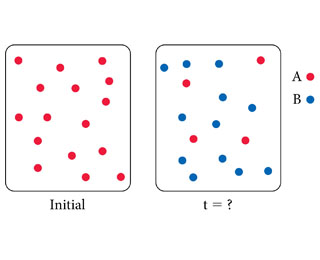# Problem: These images represent the first order reaction A initially and at some later time. The rate law for the reaction is Rate = 0.010 s-1 [A].How much time has passed between the two images?

###### Problem Details

These images represent the first order reaction A initially and at some later time. The rate law for the reaction is Rate = 0.010 s-1 [A].How much time has passed between the two images?